How Hard is Calculus?
Jun 02, 2022

HOW HARD IS CALCULUS?

Calculus, a significant subject for learning the scientific method of analysis, is quite challenging. The word calculus, in itself, brings some daunting thoughts to mind, but is calculus actually that overwhelming? To answer this question, we first have to understand what calculus is. After that, we should delve deeper into the subject to find out whether it actually is cumbersome or just perceived as such.

By the end of this article, you will learn why calculus is not just a standalone course, but an integral part of many fields of study and learn some tips to make calculus more accessible.

Brushing Up the Basics of Calculus

Many other branches of mathematics deal with static math and the calculation of perfectly still objects. Calculus, originally called infinitesimal calculus, is a branch of mathematics that deals with the rates of change. Simply put, calculus measures how everything moves and changes with time.

Calculus has two main branches: differential calculus and integral calculus. Differential calculus helps find the rate of change of a function and Inferential calculus helps determine a function if you have the rate of change for that function. Let’s explore these divisions further.

Exploring Differential Calculus

This division deals with the rate of change of one quantity concerning the difference in the other. It involves the calculation of derivatives that help solve problems of non-constant rates of change, and also finds the slope of the functions.

Suppose we have a function of x i-e ƒ (x) which is the rate of change of a function with respect to x  and ƒ '(x )  represents the derivative of x. So,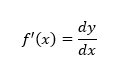For a function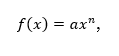the derivative ƒ (x) with respect to x is calculated as: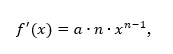and the derivative of a constant is always zero.

Let’s solve an example;

Differentiate the following function with respect to x: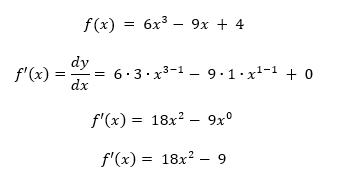When studying a subject as complex as differential calculus, students might wonder about its significance. Differential calculus has many real-life applications such as:

• Calculating profits and losses using graphical methods
• Calculating the rate of change of temperature
• Calculating the speed or distance covered by vehicles
• Deriving equations in physics

Sneak Peek into Integral CalculusIntegral calculus helps find anti-derivatives from differentiated equations. It is the reversal of differentiation i.e. it is the process of getting ƒ (x) from ƒ '(x ). It further consists of the first and second fundamental theorems of integral.

Suppose the derivative of ƒ (x) is 2x, i.e.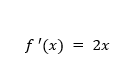Then the antiderivative of ƒ '(x ) becomes ƒ (x);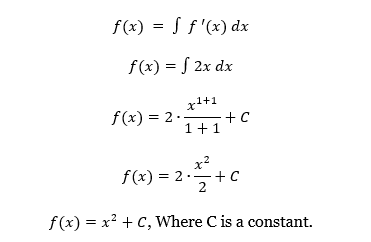In real life, integral calculus has applications in engineering mainly electrical engineering), architecture, statistics, physics, research analysis, and many other fields involving complex problem solving.

After going through the basics of calculus, let’s consider the real question at hand: why is calculus so hard?

Is Calculus Really Hard Or Are You Overthinking?

Calculus courses are the building blocks of advanced mathematics. Calculus might sound intimidating to students with a relatively weak base in middle and high school level math, especially algebra. Calculus is not the most straightforward form of math, but it is significant.

Compared to the courses taken in middle school and high school, calculus is a major jump in terms of its difficulty level. To further answer this question, we have to examine the difficulty levels of different calculus courses taught over time

Initially at high school level, students are taught pre-calculus and calculus courses. Pre-calculus helps develop basic concepts regarding sequence and series, derivatives and probabilities.

The jump from algebra to pre-calculus is quite huge, and being somewhat unrelated, increases the complexity. Students find it difficult because of the sudden, new and large number of topics. However, students with a strong knowledge of algebra tend to do better in pre-calculus and find it easier than other students.

Students often find themselves overwhelmed by this course, as it is a mixture of previously studied topics along with several new ones. You have to memorize a lot of concepts that you have not learned before, and if you do not have a strong mathematics background, you may find yourself feeling quite confused by calculus.

Calculus is rooted in the fundamentals of pre-calculus and introduces students to the concepts of differentiation and integration. To grasp these concepts, you need expertise in algebra and its concepts. High school calculus consists of mostly the computational aspect. Here students learn mostly about gradients primarily by differentiation and learn to calculate the area under the graph mainly by integration.

Since there are many calculus applications in several fields like economics, science, and engineering, it is offered at college level to complete a degree, including Calculus I, Calculus II and Calculus III courses. These are even more advanced than the calculus we study at high-school level.

Calculus I involves differentiation questions that are not too difficult to solve, but Calculus II involves a sophisticated and greater in-depth understanding of integration.; whereas, Calculus III is basically Calculus I involving vector equations in three-dimensions. There are many formulas to memorize, which most students find challenging.

At this level, these courses require strong critical thinking skills, hard work, and a thorough grasp of all the underlying concepts. It demands time, but one should not be scared by that. While students consider calculus to be a tough subject, it is a basic course for students pursuing a major in the field of mathematics.

It is possible that your seniors in college or friends you know have told you horror stories about calculus. However, it is not as disturbing as you may think, and there are trickier mathematical courses, such as topology, differential geometry, and analysis. These courses exist beyond the undergraduate level for students interested in pursuing mathematics at the advanced level.

Calculus is often compared with algebra too. Since both the subjects are seemingly dry and demand analytical thinking, they can be quite challenging. Algebra has its own quirks that we have discussed previously too, but calculus certainly wins the ‘which is harder?’ competition between these two subjects.

Calculus is more complex and advanced; plus it requires algebra as a prerequisite. Secondly, calculus involves a more advanced mathematical understanding and ability. You can think of algebra as one of the stairs used to climb towards calculus.

In conclusion then, it is not a complete exaggeration to call calculus a tough subject, but what are the different elements that contribute to its complexity? Let us delve into those in a little more depth.

Why Is Calculus Hard?

Calculus requires more hard work than basic mathematical courses. Now that we know something about the difficulty level of calculus, it is important to find out what makes it challenging.

Abstract Thinking Adds Confusion to Calculus

The subject involves abstract thinking, which requires a prerequisite of several basic concepts, and critical thinking, which is different from the concepts taught in other subjects.

Students who struggle with calculus are primarily those with a weak understanding of the fundamental courses. Calculus is a branch of mathematics, and mathematics is sequential. You would not think of learning to divide or multiply before learning to add or subtract, right?  Similarly, you will struggle to excel in calculus if your fundamentals of mathematics are weak.

In order to excel at any level, it is important to master the introductory concepts. According to a survey of over 200 people by Tim Stadler, 68.9% of calculus students did not consider calculus a hard course. That, of course, means the remaining 31.1% agreed it is. When asked why they thought it was hard, they responded that it was dependent on the student's lack of interest, the inability of the instructor teaching the course, or the lack of fundamental knowledge of algebra.

Calculus demands commitment. You cannot treat it the same as all the other theoretical subjects that can be read and understood with little effort. To do well in calculus, you have to devote time and effort beyond regular classes. To expect to excel at calculus without considering the advanced status of this course would be wrong.

Why Is Calculus So Important To Study?

Calculus is a very broad subject with vast applications in several fields, which is why calculus is taught in both STEM and NON-STEM courses. For example, ‘probability’, a concept of calculus is used in computer science. It would help if you had an understanding in medical sciences as well for modelling of diseases.

To provide a better user experience, computer search engines also use calculus to create an algorithm to generate more efficient results. Calculus formulas are used to measure the rate of growth in a population and also the rates of chemical reactions in an experiment.

Moreover, calculus is used by scientists in developing discoveries and inventions in engineering fields. Calculus also helps a great deal to understand the miracles of scientific analysis methods. You study how things change and how motion affects different situations in calculus. Since both these elements are used in scores of subjects and areas, calculus applications are limitless.

So far we have established that calculus, if not as hard as climbing Mount Everest, is at least close enough to that challenge. That said, if you are going to study it, why not find out some ways to make it easier? Let us discuss those next.

What Can You Do To Improve?

Calculus is a fascinating part of mathematical science and has several exciting implications in the real world, as mentioned above. Suppose this stirred your interest in calculus and you are still thinking about ways to overcome the fear associated with the difficulty level of calculus. In that case, we might have a few solutions for you.

First of all, you have to recognize the reason why you find calculus hard. It may be due to the following:

• Not putting enough time into studying
• Lack of interest
• Thinking that it is like other theoretical and less time-consuming subjects
• Lack of practice and homework problems
• Not paying attention in class and taking quality notes
• Considering calculus as something unimportant.

Once you have realized the issue, you have to address the following:

• Fill the cracks in your fundamental mathematical knowledge
• Try to solve questions on your own and before the class starts
• Take thorough notes
• Practice more and more every day
• Brush up your concepts with the help of internet videos
• Practice daily instead of piling up work for the next day
• Memorize the tips and tricks at your fingertips, but also read into the insights and try to grasp the concepts.

There is Light At The End of the Tunnel2

Mathematics is a vast subject and is the mother of all sciences consisting of various subdivisions and courses. Calculus forms the basis of advanced mathematics. Its inclusion in STEM and NON-STEM fields further stresses its importance.

Calculus has numerous applications in almost all fields and is used to study anything undergoing change. As a student, you may find it hard, but this difficulty is rooted in your weaker concepts of mathematics too, so brush up on them first. If you aspire to pursue a degree in advanced mathematics, calculus is a fundamental course.

Mathematics, a sequential science, consists of steps wherein you need to carry out one calculation to move to the next step. The same applies to calculus. To better understand calculus at any stage, it is essential to have a strong base in the fundamental concepts of algebra, pre-calculus, and geometry.

Yes, calculus is a hard subject, but if you are committed to it, and work hard, you will ace it. Attending routine calculus lectures is just not enough to master calculus. You need to revise your previous concepts, brush up on your knowledge and understanding of algebra, and work on your weak areas in calculus daily. If you do that persistently, you can become a pro at calculus.

 Tim Statler is a Computer Science student at Governors State University and the creator of Comp Sci Central.

Popular Posts24houranswers.com Parker Paradigms, Inc 5 Penn PLaza, 23rd Floor New York, NY 10001
SUBJECTS COVERED